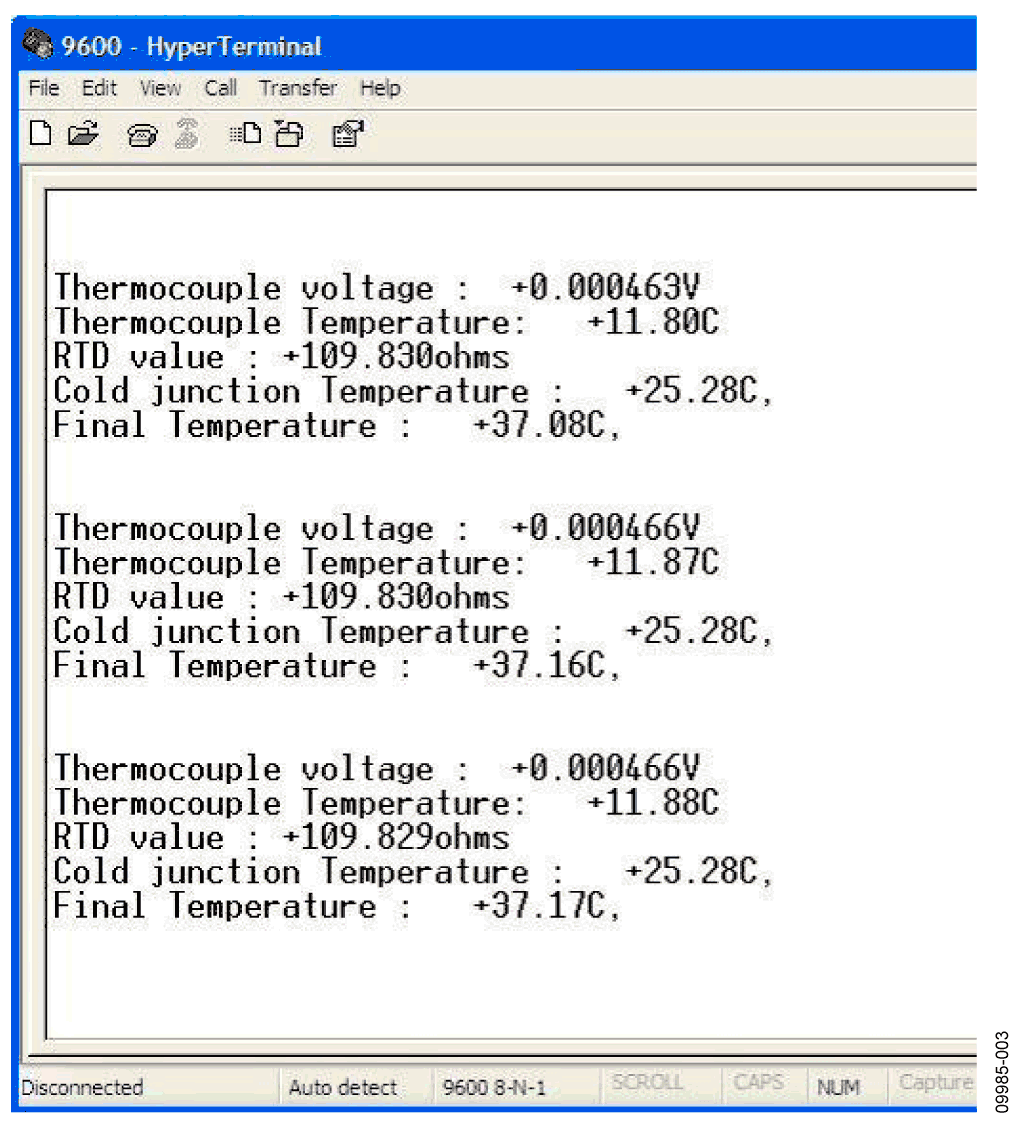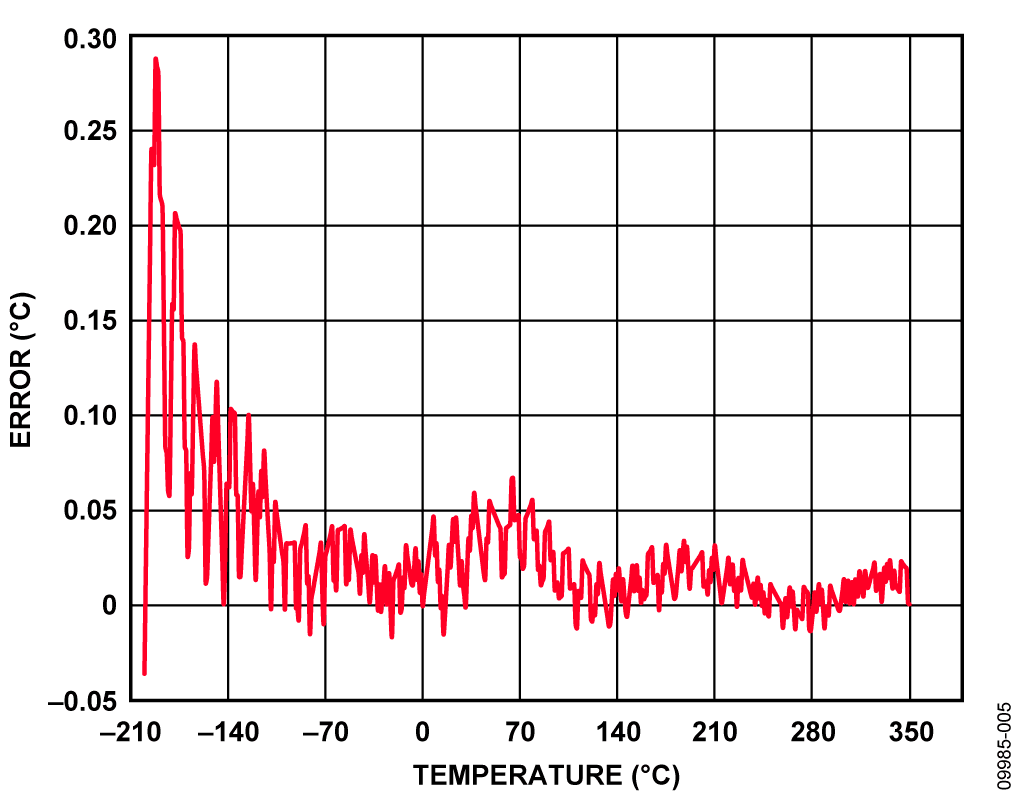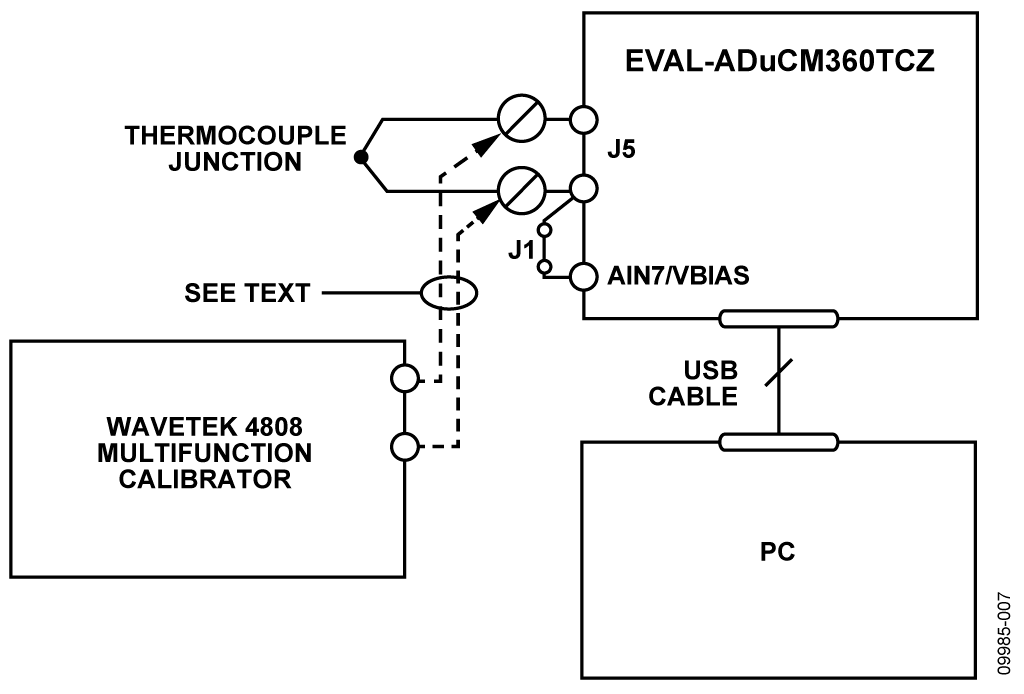Evaluation Hardware \$65.00# Overview

## Design Resources

### Design & Integration Files

• Schematic
• Bill of Materials
• Gerber Files
• Assembly Drawing

### Evaluation Hardware

Part Numbers with "Z" indicate RoHS Compliance. Boards checked are needed to evaluate this circuit

• EVAL-CN0221-EB1Z (\$65.00) USB-Based Temperature Monitor Using the ADuCM360 Precision Analog Microcontroller and an External Thermocouple

## Features & Benefits

• Typical temperature range of -200 C to +400 C
• Precision Cortex M3 analog microcontroller with 24-bit sigma-delta ADC
• T-type thermocouple measurement system with cold junction compensation
• Single chip solution

# Circuit Function & Benefits

This circuit uses the ADuCM360/ADuCM361 precision analog microcontroller in an accurate thermocouple temperature monitoring application. The ADuCM360/ADuCM361 integrates dual 24-bit sigma-delta (Σ-Δ) analog-to-digital converters (ADCs), dual programmable current sources, a 12-bit digital-to-analog converter (DAC), and a 1.2 V internal reference, as well as an ARM Cortex-M3 core, 128 kB flash, 8 kB SRAM, and various digital peripherals such as UART, timers, SPIs, and I2C interfaces.

In the circuit, the ADuCM360/ADuCM361 is connected to a thermocouple and a 100 Ω platinum resistance temperature detector (RTD). The RTD is used for cold junction compensation.

In the source code, an ADC sampling rate of 4 Hz is chosen. When the ADC input programmable gain amplifier (PGA) is configured for a gain of 32, the noise-free code resolution of the ADuCM360/ ADuCM361 is greater than 18 bits.Figure 1. ADuCM360/ADuCM361 as a Temperature Monitor Controller with a Thermocouple Interface (Simplified Schematic, All Connections Not Shown)

# Circuit Description

• A 24-bit Σ-Δ ADC with a PGA set for a gain of 32 in the software for the thermocouple and RTD. The ADC1 was switched continuously between sampling the thermocouple and the RTD voltages.
• Programmable excitation current sources for forcing a controlled current through the RTD. The dual current sources are configurable in from 0 μA to 2 mA. For this example, a 200 μA setting was used to minimize the error introduced by the RTD self-heating.
• An internal 1.2 V reference for the ADC in the ADuCM360/ ADuCM361. It measures the thermocouple voltage; the internal voltage reference was used due to its precision.
• An external voltage reference for the ADC in the ADuCM360/ADuCM361. It measures the RTD resistance; a ratiometric setup was used where an external reference resistor (RREF) was connected across the external VREF+ and VREF− pins.
• A bias voltage generator (VBIAS). The VBIAS function was used to set the thermocouple common-mode voltage to AVDD/2.
• The ARM Cortex-M3 core. The powerful 32-bit ARM core with integrated 128 kB flash and 8 kB SRAM memory runs the user code that configures and controls the ADC, processes the ADC conversions from the RTD, and controls the communications over the UART/USB interface.
• The UART was used as the communication interface to the host PC.
• Two external switches are used to force the part into its flash boot mode. By holding SD low and toggling the RESET button, the ADuCM360/ADuCM361 enters boot mode instead of normal user mode. In boot mode, the internal flash can be reprogrammed through the UART interface.

Both the thermocouple and the RTD generate very small signals; therefore, a PGA is required to amplify those signals.

The thermocouple used in this application is a Type T (copper-constantan) that has a temperature range of −200°C to +350°C. Its sensitivity is approximately 40 μV/°C, which means that the ADC in bipolar mode, with a PGA gain of 32, can cover the entire temperature range of the thermocouple.

The RTD was used for cold junction compensation. The particular one used in this circuit was a platinum 100 Ω RTD, Enercorp PCS 1.1503.1. It is available in a 0805, surface-mount package. This RTD has a temperature variation of 0.385 Ω/°C.

Note that the reference resistor, RREF, should be a precision 5.6 kΩ (±0.1%).

The USB interface to the ADuCM360/ADuCM361 is implemented with an FT232R UART to USB transceiver, which converts USB signals directly to the UART.

In addition to the decoupling shown Figure 1, the USB cable itself must have a ferrite bead for added EMI/RFI protection. The ferrite beads used in the circuit were Taiyo Yuden, #BK2125HS102-T, which have an impedance of 1000 Ω at 100 MHz.

Construct the circuit on a multilayer printed circuit board (PCB) with a large area ground plane. Use proper layout, grounding, and decoupling techniques to achieve optimum performance (see Tutorial MT-031, Grounding Data Converters and Solving the Mystery of "AGND" and "DGND," Tutorial MT-101, Decoupling Techniques, and the ADuCM360TCZ Evaluation Board layout).

The PCB used for evaluating this circuit is shown in Figure 2.Figure 2. EVAL-ADuCM360TCZ Board Used for this Circuit

Code Description

The link to the source code used to test the circuit can be found in the CN0221 Design Support Package located at http://www.analog.com/CN0221-DesignSupport.

The UART is configured for a baud rate of 9600, 8 data bits, no parity, and no flow control. If the circuit is connected directly to a PC, a communication port viewing application, such as a HyperTerminal, can be used to view the results sent by the program to the UART, as shown in Figure 3.Figure 3. Output of HyperTerminal Communication Port Viewing Application

To get a temperature reading, measure the temperature of the thermocouple and the RTD. The RTD temperature is converted to its equivalent thermocouple voltage via a look-up table (see the ISE, Inc., ITS-90 Table for Type T Thermocouple). These two voltages are added together to give the absolute value at the thermocouple.

First, the voltage measured between the two wires of the thermocouple (V1). The RTD voltage is measured, converted to a temperature via a look-up table, and then, this temperature is converted to its equivalent thermocouple voltage (V2). V1 and V2 are then added to give the overall thermocouple voltage, and this is then converted to the final temperature measurement.Figure 4. Error When Using Simple Linear Approximation

Initially, this was done using a simple linear assumption that the voltage on the thermocouple was 40 μV/°C. It can be seen from Figure 4 that this gives an acceptable error only for a small range, around 0°C. A better way of calculating the thermocouple temperatures is to use a six-order polynomial for the positive temperatures and a seventh-order polynomial for the negative temperatures. This requires mathematical operations that add to computational time and code size. A suitable compromise is to calculate the respective temperatures for a fixed number of voltages. These temperatures are stored in an array, and values in between are calculated using a linear interpolation between the adjacent points. It can be seen from Figure 5 that the error is drastically reduced using this method. Figure 5 gives the algorithm error using ideal thermocouple voltages.Figure 5. Error When Using Piecewise Linear Approximation Using 52 Calibration Points and Ideal Measurements

Figure 6 shows the error obtained when using ADC1 on the ADuCM360 to measure 52 thermocouple voltages over the full thermocouple operating range. The overall worst-case error is <1°C.Figure 6. Error When Using Piecewise Linear Approximation Using 52 Calibration Points Measured by ADuCM360/ADuCM361

The RTD temperature is calculated using lookup tables and is implemented for the RTD the same way as for the thermocouple. Note that the RTD has a different polynomial describing its temperatures as a function of resistance.

For details on linearization and maximizing the performance of the RTD, refer to Application Note AN-0970, RTD Interfacing and Linearization Using an ADuC706x Microcontroller.

# Common Variations

The ADP1720 regulator can be replaced with the ADP120, which has the same operating temperature range (−40°C to +125°C) and consumes less power (typically 35 μA vs. 70 μA) but has a lower maximum input voltage. Note that the ADuCM360/ADuCM361 can be programmed or debugged via a standard serial wire interface.

For a standard UART to RS-232 interface, the FT232R transceiver can be replaced with a device such as the ADM3202, which requires a 3 V power supply. For a wider temperature range, a different thermocouple can be used, such as a Type J. To minimize the cold junction compensation error, a thermistor can be placed in contact with the actual cold junction instead of on the PCB.

Instead of using the RTD and external reference resistor for measuring the cold junction temperature, an external digital temperature sensor can be used. For example, the ADT7410 can connect to the ADuCM360/ADuCM361 via the I2C interface.

For more details on cold junction compensation, refer to Signal Conditioning, Analog Devices, Chapter 7, “Temperature Sensors.”

If isolation between the USB connector and this circuit is required, the ADuM3160/ADuM4160 isolation devices must be added.

# Circuit Evaluation & Test

To test and evaluate the circuit, the thermocouple measurements and the RTD measurements were evaluated separately.

Thermocouple Measurement Test

The basic test setup is shown in Figure 7. The thermocouple is connected to J5, and Jumper J1 must be installed to allow the AIN7/VBIAS pin to set the thermocouple common-mode voltage. The circuit board receives its power from the USB connection to the PC.

Two methods were used to evaluate the performance of the circuit. Initially, the circuit was tested with the thermocouple attached to the board and it was used to measure the temperature of an ice bucket. Then, it was used to measure the temperature of boiling water.

A Wavetek 4808 Multifunction Calibrator was used to fully evaluate the error, as shown in Figure 4 and Figure 6. In this mode, the thermocouple was replaced with the calibrator as the voltage source, as shown in Figure 7. To evaluate the entire range of a Type T thermocouple, the calibrator was used to set the equivalent thermocouple voltage at 52 points between −200°C to +350°C for the negative and positive ranges of the T-type thermocouple (see the ISE, Inc., ITS-90 Table for Type T Thermocouple).

To evaluate the accuracy of the lookup algorithm, 551 voltage readings, equivalent to temperatures in the range of −200°C to +350°C spaced at +1°C, were passed onto the temperature calculation functions. Errors were calculated for the linear method and the piecewise linear approximation method as isshown in Figure 4 and Figure 5.Figure 7. Test Setup Used to Calibrate and Test the Circuit Over Full Thermocouple Output Voltage Range

RTD Measurement Test

To evaluate the RTD circuit and linearization source code, the RTD on the board was replacement with an accurate, adjustable resistance source. The instrument used was the 1433-Z Decade Resistor. The RTD values are from 90 Ω to 140 Ω, which represents an RTD temperature range of −25°C to +114°C.

The test setup circuit is shown in Figure 8, and the error results for the RTD tests are shown in Figure 9.Figure 8. Test Setup for Measuring RTD ErrorFigure 9. Error in °C of RTD Measurement Using Piecewise Linearization Code and ADC0 Measurements